ConceptDraw
DIAGRAM 14

# ConceptDraw DIAGRAM UML Diagrams with ConceptDraw DIAGRAM

Unified Modeling Language (UML) is a standardized general-purpose modeling language in the field of software engineering.

When creating UML diagrams it is highly recommended that a special UML modeling or design tool be used. ConceptDraw DIAGRAM is a great UML modeling and design tool that enables you to quickly and easily generate all types of UML diagrams. ConceptDraw DIAGRAM includes a large set of templates for UML design, UML diagram samples, and a UML modeling reference guide.

ConceptDraw DIAGRAM offers a large collection of industry-standard UML object libraries for all types of UML diagrams:

• Class Diagram: Objects, Packages, Subsystems, and Models
• UML Packages Diagram: UML Use Case Diagram, UML Class Diagram
• UML Deployment Diagram, UML State Diagram, UML Collaboration Diagram
• UML Sequence Diagram, UML Activity Diagram, UML State Diagram

UML Modeling Tool Samples:

 UML Class Diagram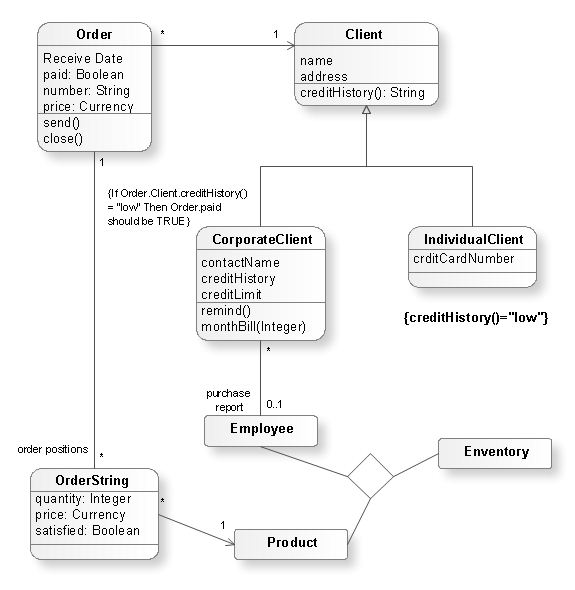UML Sequence Diagram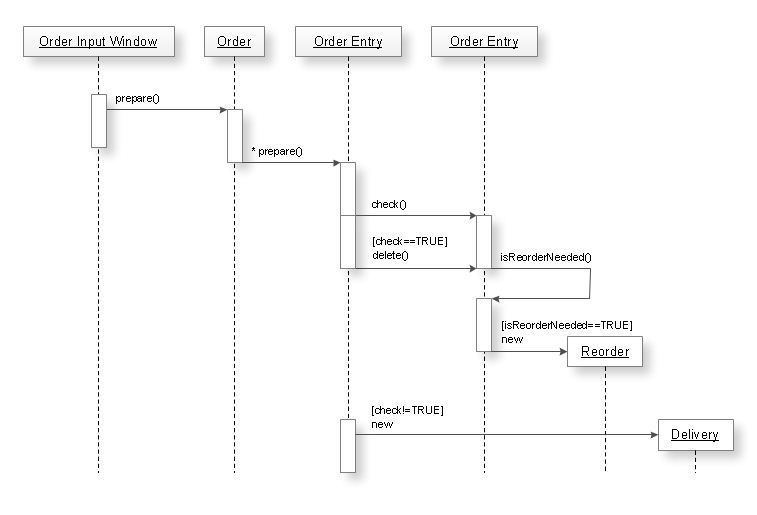UML Use Case Diagram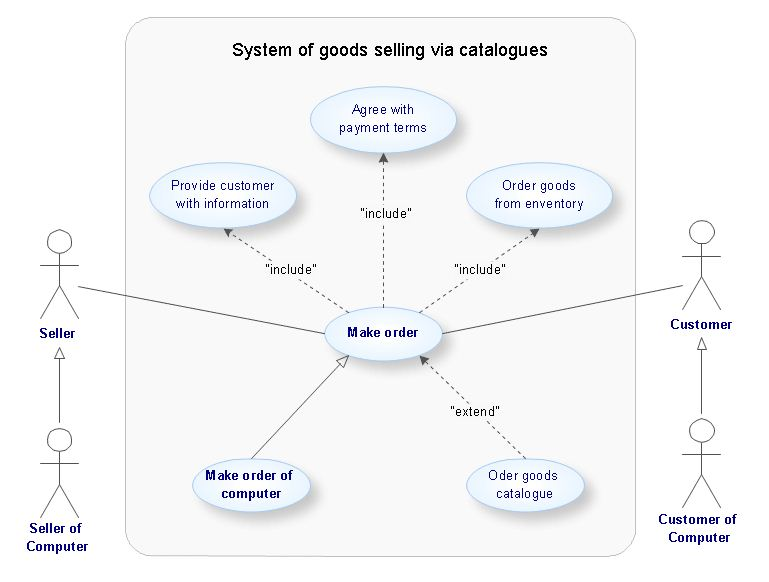### Sequence Diagram for Cloud Computing →

One of the ways effectively visualize what is a Cloud computing or Cloud computing architecture, is to create the Sequence diagram for Cloud computing. The ConceptDraw DIAGRAM diagramming and vector drawing software enhanced with Cloud Computing Diagrams solution from the Computers and Network area of ConceptDraw Solution Park will help you design all desired types of diagrams related with cloud computing.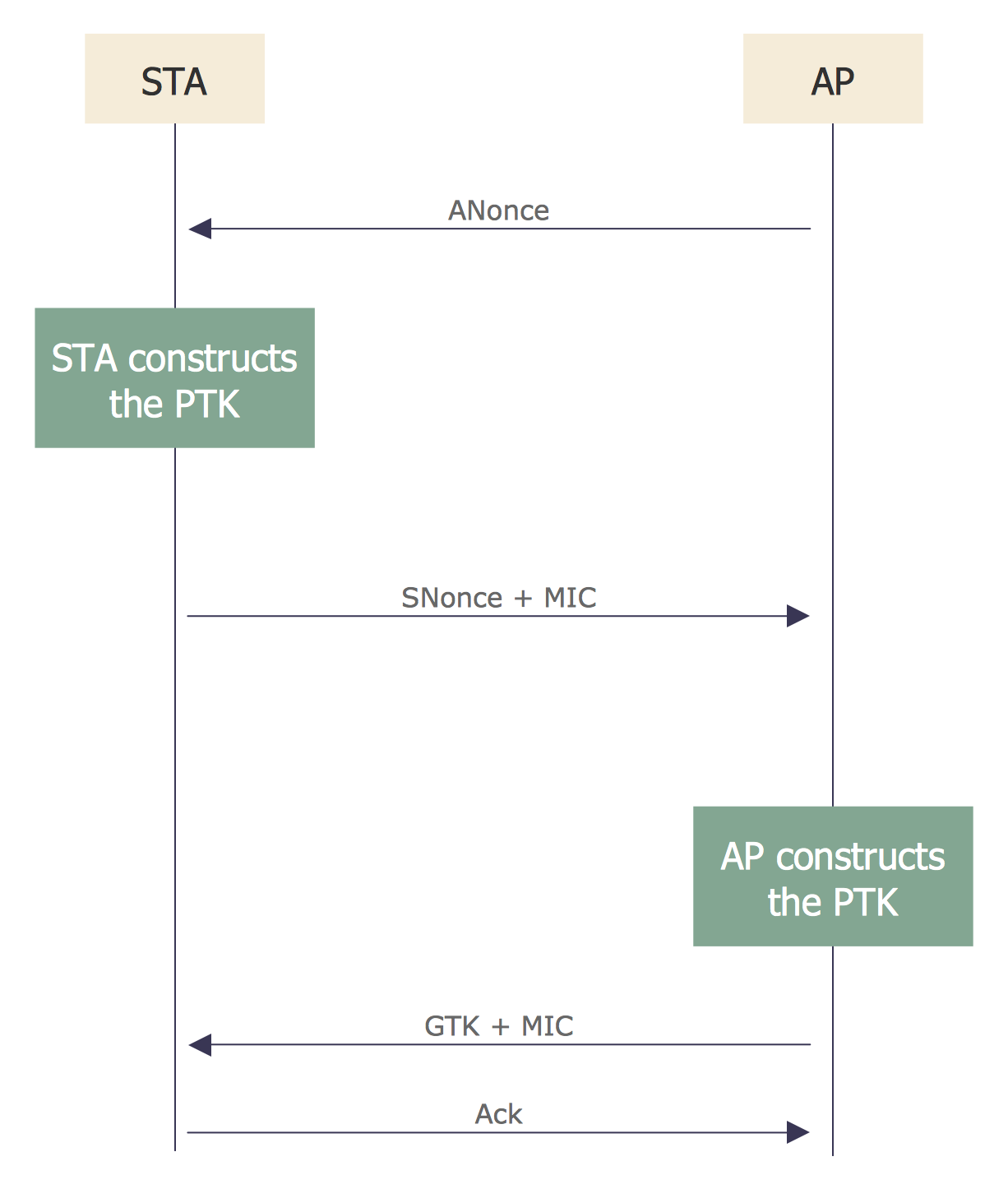Picture: Sequence Diagram for Cloud Computing
Related Solution:

### Sequence Diagram Tool →

ConceptDraw DIAGRAM diagramming and vector drawing software as a sequence diagram tool provides the Rapid UML Solution from the Software Development Area that contains the UML Sequence library.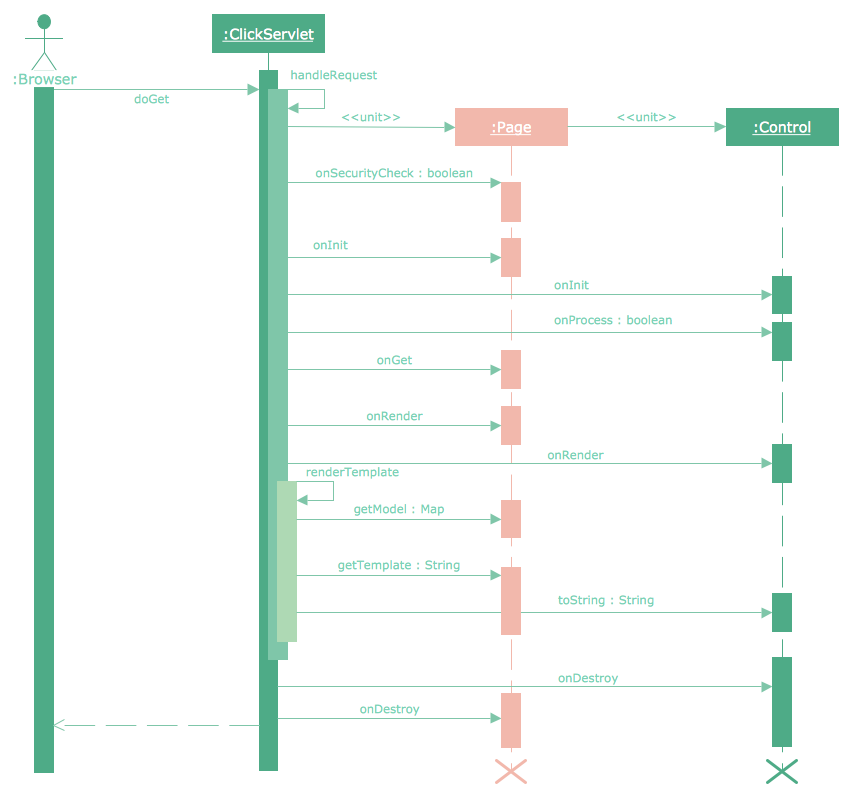Picture: Sequence Diagram Tool
Related Solution:

### Online Diagram Tool →

ConceptDraw DIAGRAM diagramming and vector drawing software extended with Rapid UML Solution from the Software Development Area is a powerful online diagram tool that will help you design any types of UML diagrams simply and fast.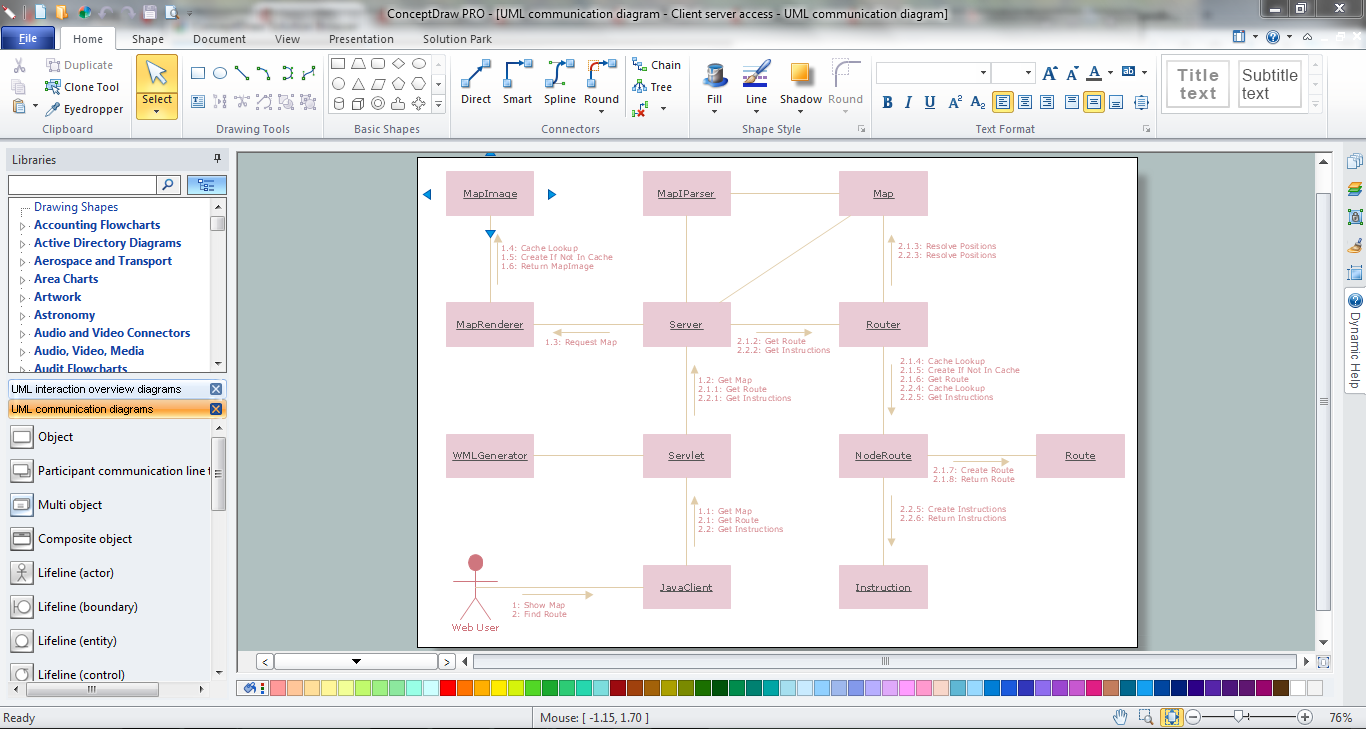Picture: Online Diagram Tool
Related Solution:

### Functional Block Diagram →

You need design the Functional Block Diagram and dream to find the useful tools to draw it easier, quickly and effectively? ConceptDraw DIAGRAM offers the Block Diagrams Solution from the "Diagrams" Area which will help you!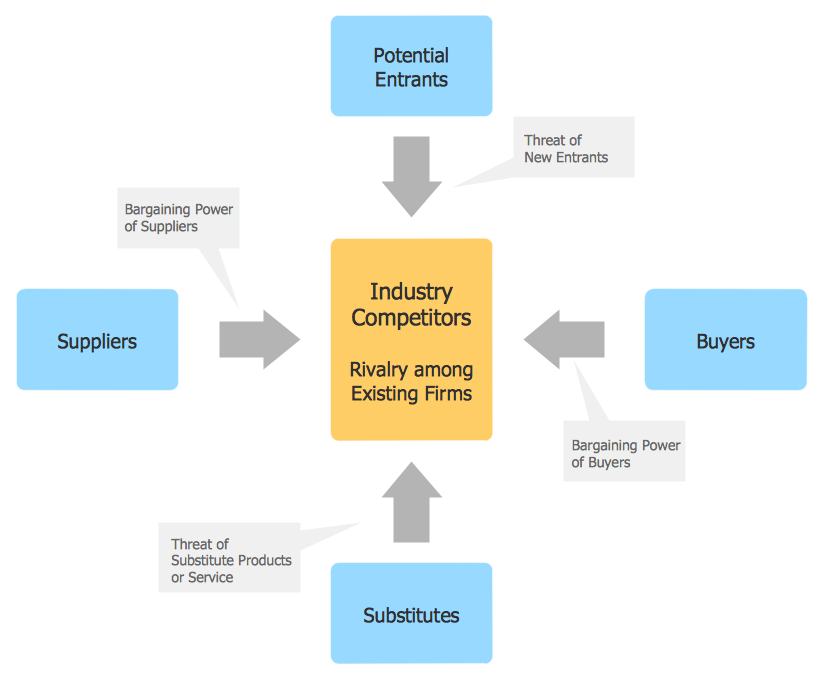Picture: Functional Block Diagram
Related Solution:

### Memory Object Diagram →

The vector stencils library UML Object Diagram from the solution Rapid UML contains UML specific symbols such as object symbol, association symbols, link symbols, operation symbols for ConceptDraw DIAGRAM diagramming and vector drawing software. The Rapid UML solution is contained in the Software Development area of ConceptDraw Solution Park.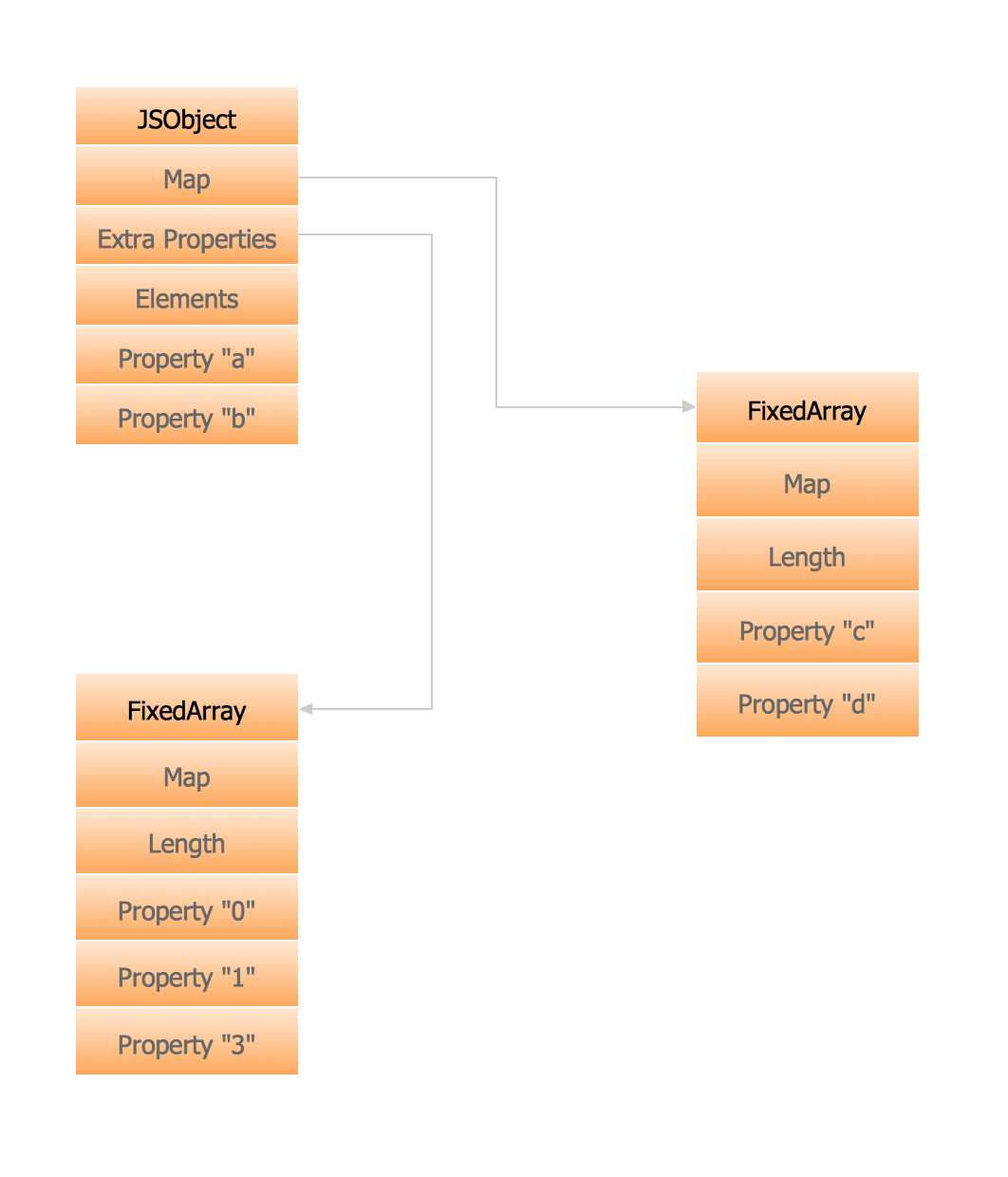Picture: Memory Object Diagram
Related Solution:

### Unified Modeling Language Diagram →

The UML Diagrams are widely used by software developers and software engineers. They can draw the UML Diagrams manually on the paper, but it is incredibly convenient to use the special UML diagramming software. The UML diagramming software allows you to draw the UML Diagrams in a few minutes.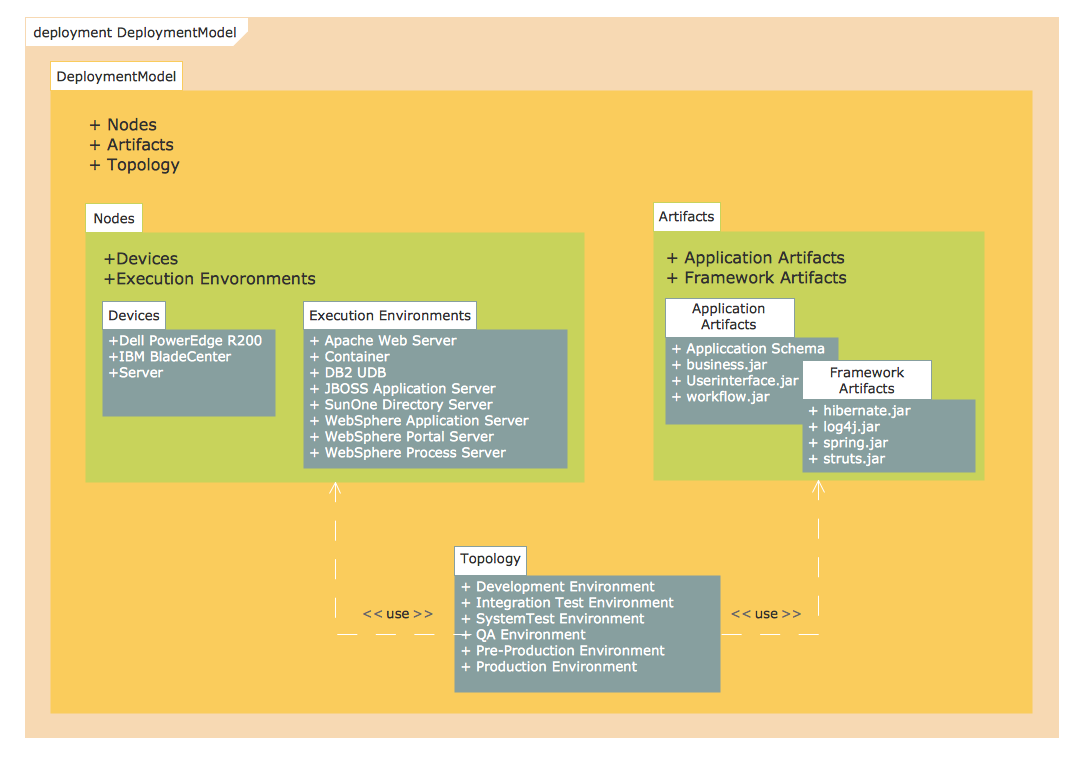Picture: Unified Modeling Language Diagram
Related Solution:

### UML Diagram for Mac →

This sample was created on the Mac in ConceptDraw DIAGRAM diagramming and vector drawing software using the UML State Machine Diagram library of the Rapid UML Solution from the Software Development area of ConceptDraw Solution Park.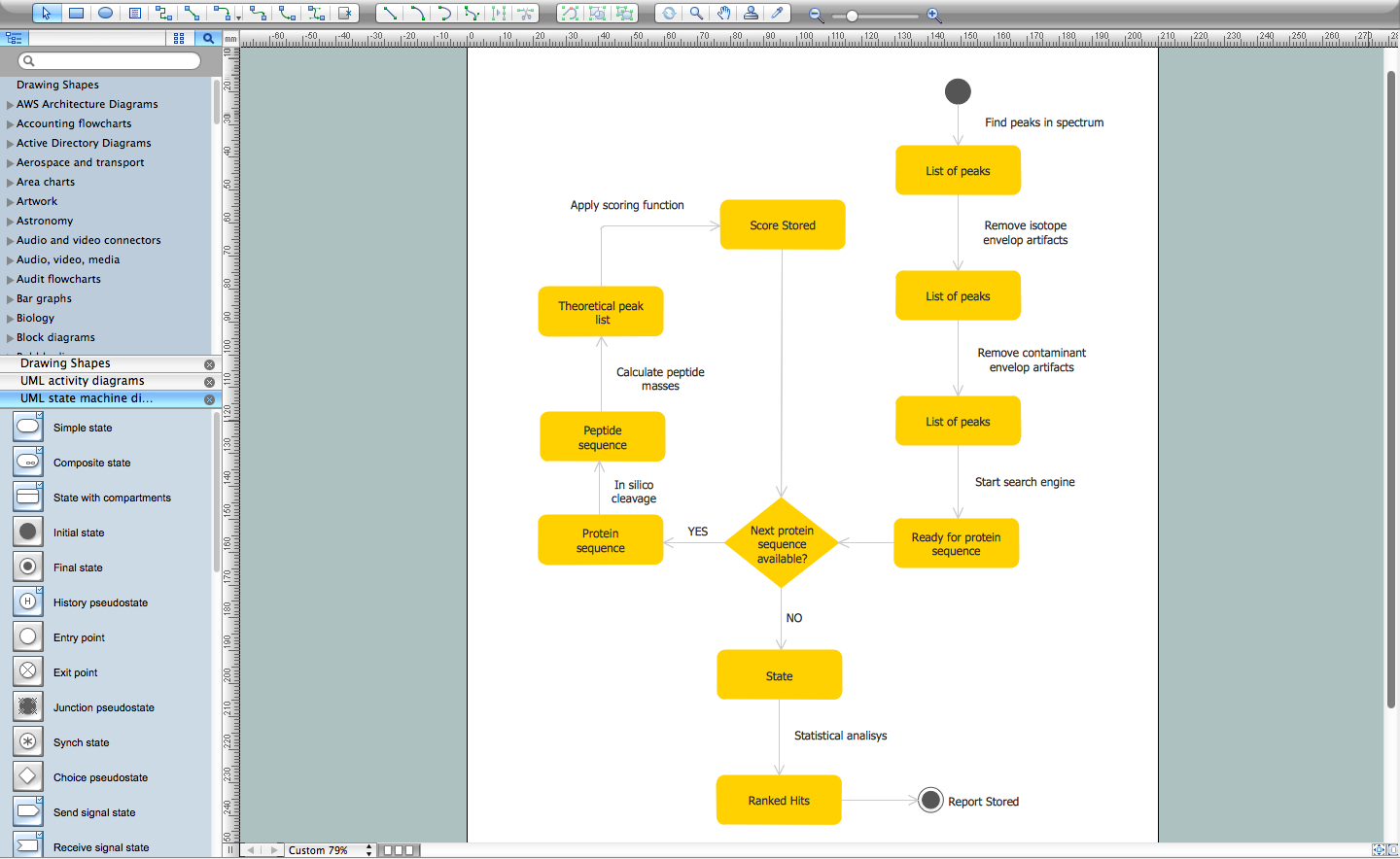Picture: UML Diagram for Mac
Related Solution:
ConceptDraw
DIAGRAM 14